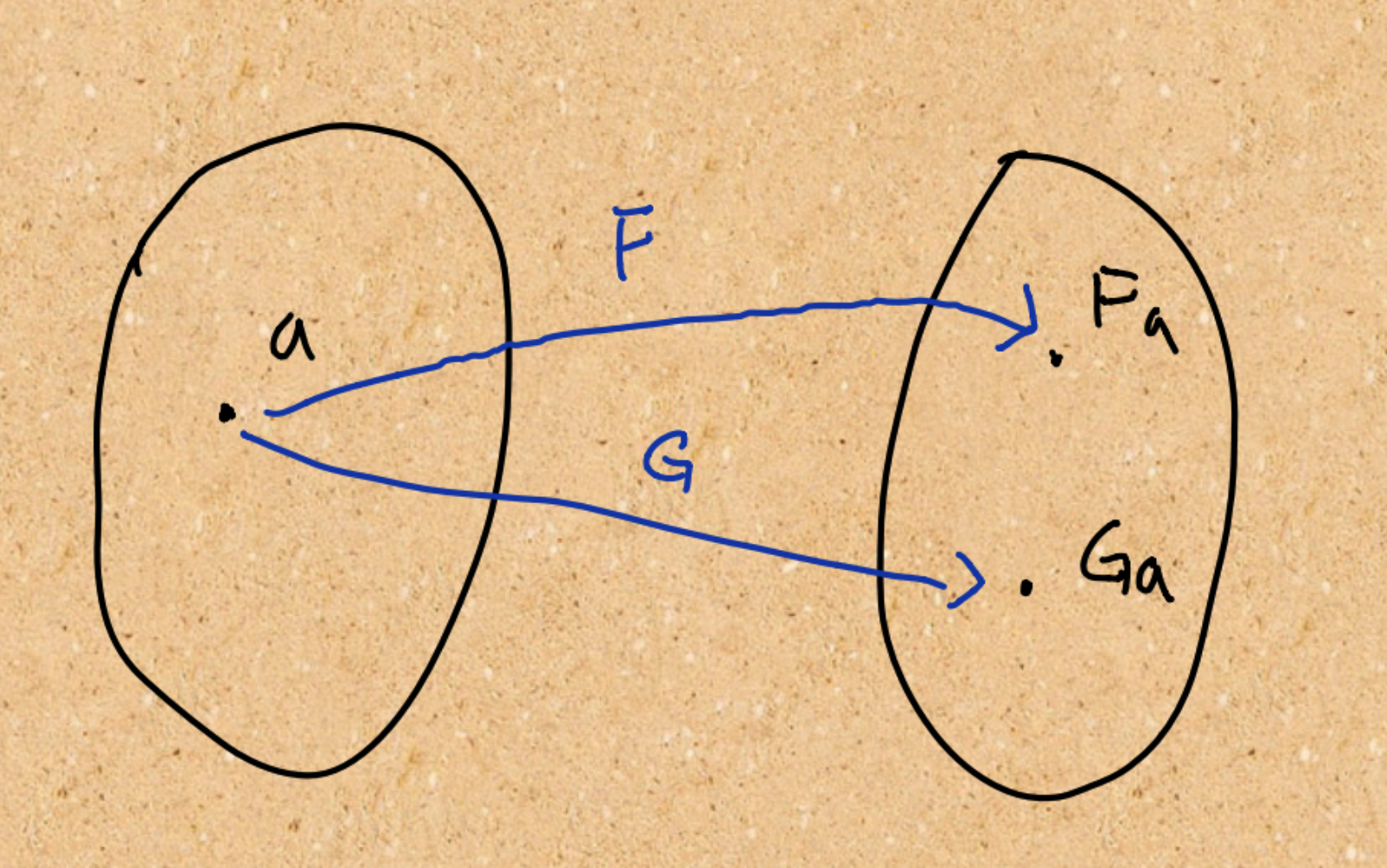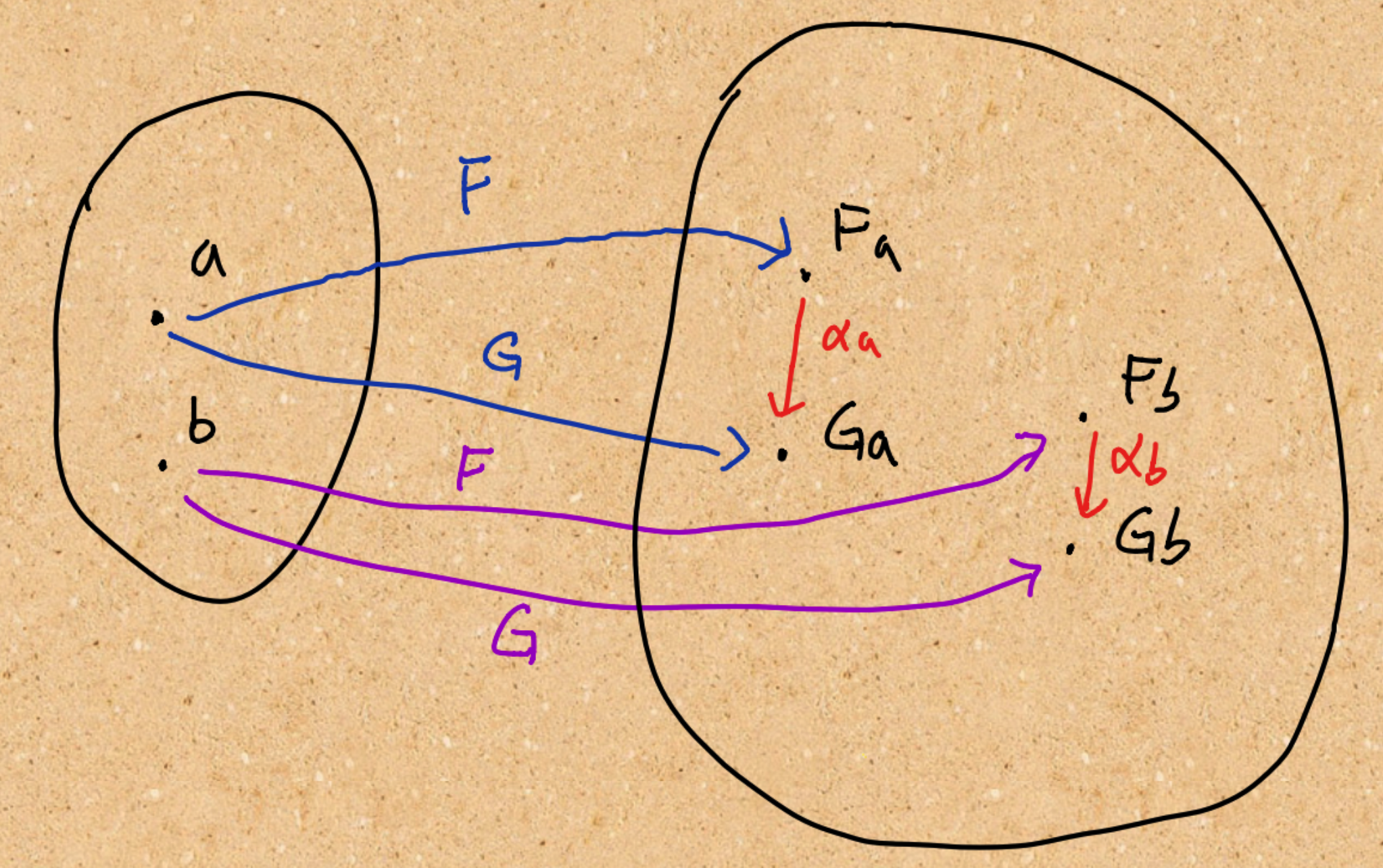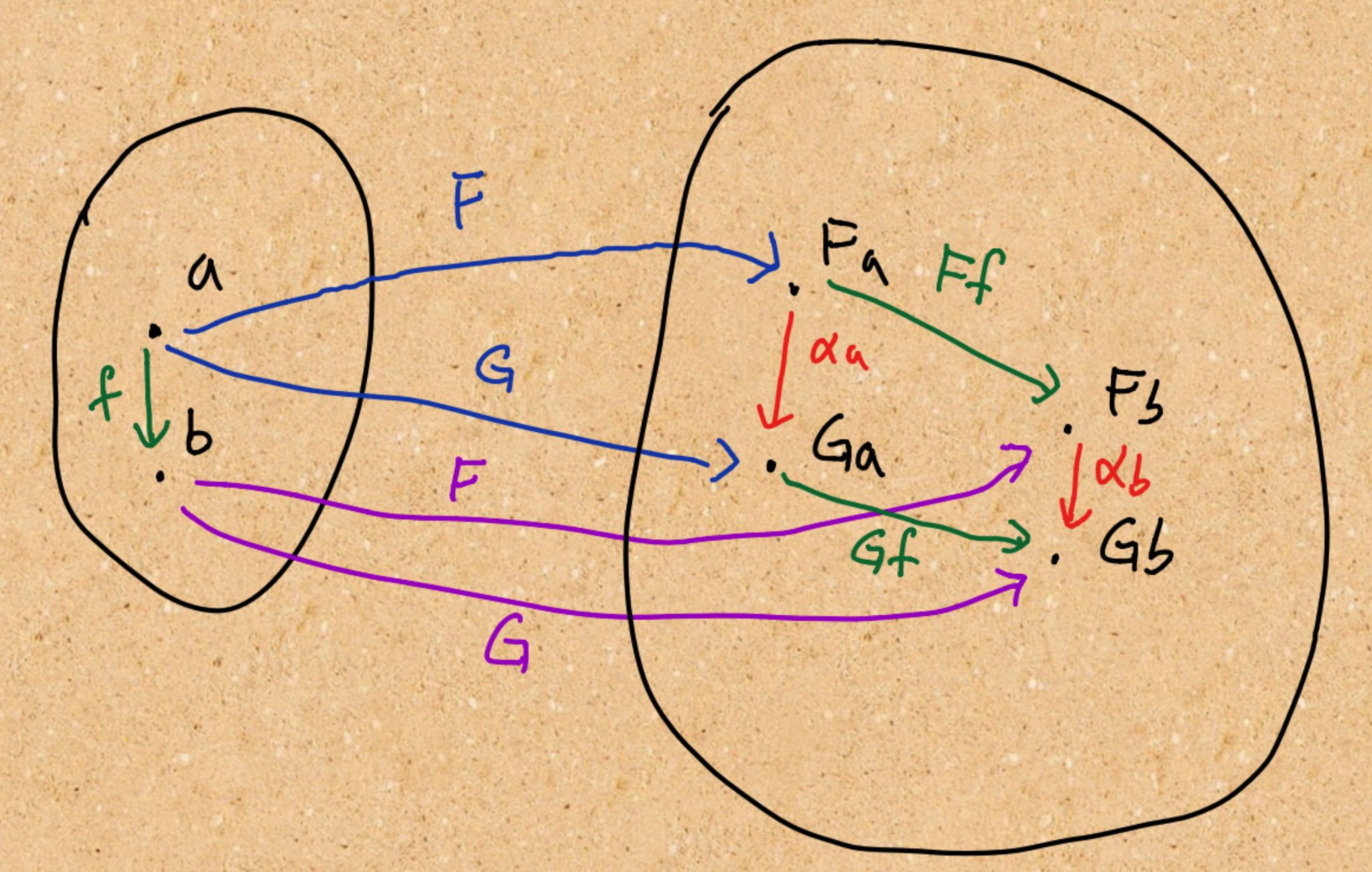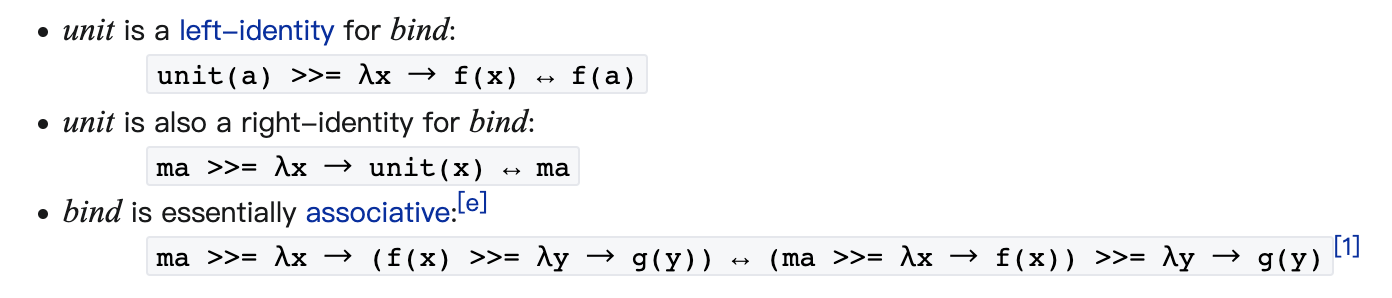#DAY 23
0
Software Development

# Definition# Code sample

``````class LinkedList<T> {
}
``````

``````// 推導   AlphaB。Ff
//
// f     -> x + 1
val a = 1
// 
// 
val Ffa: LinkedList<Int> = Fa.map { it + 1}
// Some(2)
``````

``````// 推導   Gf。AlphaA
//
// G      -> Maybe
// f      -> x + 1
val a = 1
// 
// Some(1)
// Some(2)
val Gf_alphaA: Maybe<Int> = alphaA.map{ it + 1}
``````

``````// 推導   AlphaB。Ff
//
// f     -> x + 1
// []
// []
val Ffa: LinkedList<Int> = Fa.map { it + 1}
// None
``````

``````// 推導   Gf。AlphaA
//
// G      -> Maybe
// f      -> x + 1
// []
// None
// None
val Gf_alphaA: Maybe<Int> = alphaA.map{ it + 1}
``````

# 小結